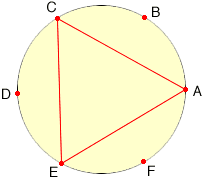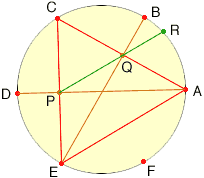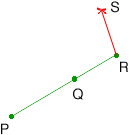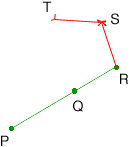Quandaries and Queries We were wondering what the instructions/formula, step-by-step, is to make a pentagon. Name: Mary Level: middle (7-9) Who: Librarian Thank you, Mary Hi Mary, I assume that you are looking for a "ruler and compass" construction. With a compass draw a circle. Choose a point A on the circle. With the same radius as the circle, put the point of the compass at A and draw an arc intersecting the circle at B. With the same radius and centre B draw and arc that intersect the circle at C. Continue to construct points A, B, C, D, E and F on the circle. Join A, C and E to form an equilateral triangle.Draw the line segment AD intersecting CE at P. Draw the line segment EB intersecting AC at Q. P is the midpoint of CE and Q is the midpoint of AC. Join P and Q and extend this line to meet the circle at R.We are now going to construct a pentagon with side length QR and diagonal length PQ. Put the compass at R and with radius QR draw an arc. With the compass at Q and radius PQ draw an arc to intersect the first arc at S. Join RS.Put the compass at S and with radius QR draw an arc. With the compass at R and radius PQ draw an arc to intersect the first arc at T. Join ST.Repeat once more with the compass at T to construct the point U and then QRSTU is a pentagon. An amateur mathematician named George Odom discovered the construction and sent it to the famous Canadian geometer H.S.M. Coxeter. Coxeter, amazed that it had not been discovered long ago, submitted it in Odom's name to the American Mathematical Monthly where it appeared in the mid 1980's. The featured solution consisted of a picture accompanied by one word: "Behold!" Chris and Penny Go to Math Central